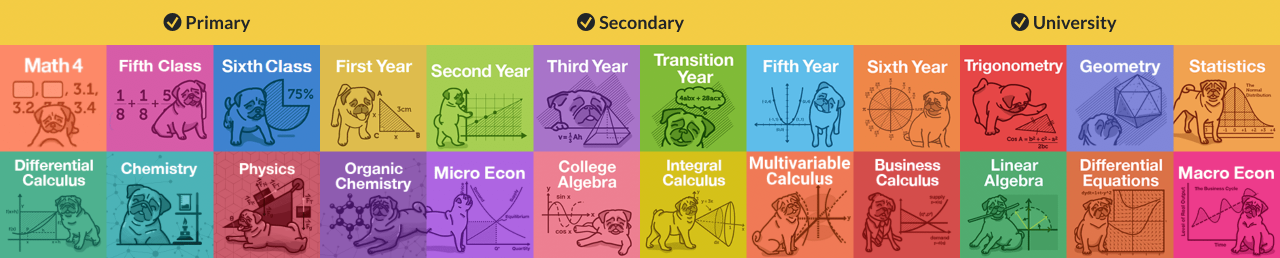# Differential Equations Help & Practice

We've got you covered with our complete help for any Ordinary Differential Equations (ODE) courses, whether you are a math major, engineering major or in any fields that are related to math and sciences.

Our comprehensive lessons on differential equations cover help on topics like First order differential equation, Second order differential equation, Separable differential equations, Laplace transforms, and a lot more. Learn the concepts with our video tutorials made by our differential equations tutors that show you step-by-step solutions to even the hardest differential equations problems. Then, strengthen your understanding with tons of differential equations practice.

All our lessons are taught by experienced Differential Equations teachers. Let's finish your homework in no time, and ACE that final.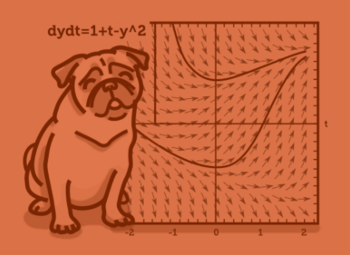#### All in One Place

Everything you need for JC, LC, and college level maths and science classes.#### Learn with Ease

We’ve mastered the national curriculum so that you can revise with confidence.#### Instant Help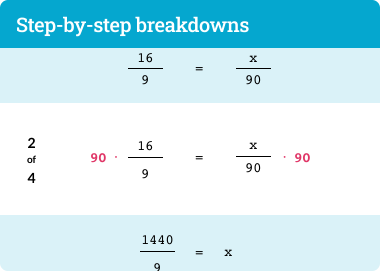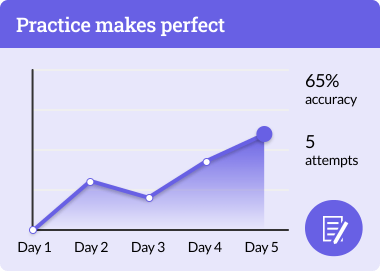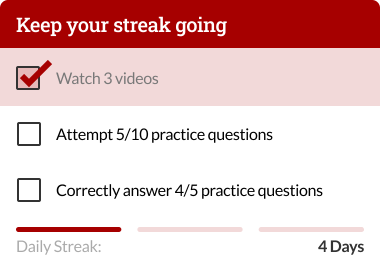1. ##### 1Introduction to Differential Equations ?
1. 1.1Introduction to differential equations
4. ##### 4Laplace Transforms
• ###### Should I take Linear Algebra before Differential Equations?

Differential equations and Linear algebra are more or less independent of each other. Some schools might recommend students to take Linear algebra first, but it is not necessary. Sometimes students even take differential equations and linear algebra concurrently.

• ###### What should I take before taking Differential Equations?

The pre-requisites of this course should be Calculus 1 (a.k.a. Differential calculus or Differentiation) and Calculus 2 (a.k.a. Integral calculus or Integration) because ODE involves a lot of integration calculations. If you need to review on materials learned from previous semesters, no worries! Your StudyPug subscription gives you unlimited access to all math help in all courses

• ##### Students and parents love our maths help
###### But don't take our word for it…
•Carson E.
parent

When we saw our son's grades we looked online for a convenient, affordable and effective solution. StudyPug has been a great investment.

•Jason G.
high school senior

This website saved my butt last semester. I am using it againthis semester. Dennis is the best online tutor... I also like that I can watch videos over and over until I really understand the concept. If you want to save time, sign up...it's only ten bucks and it saved me hours of study time. Thanks, Dennis!

•Aaron M.
high school student

I get a whole library of videos that covers everything from basic to complex mathematics for a very low monthly price. Excellent videos, easy-to-understand and most of all they work. My math test results are 10 points higher than last semester.

• See all our Testimonials